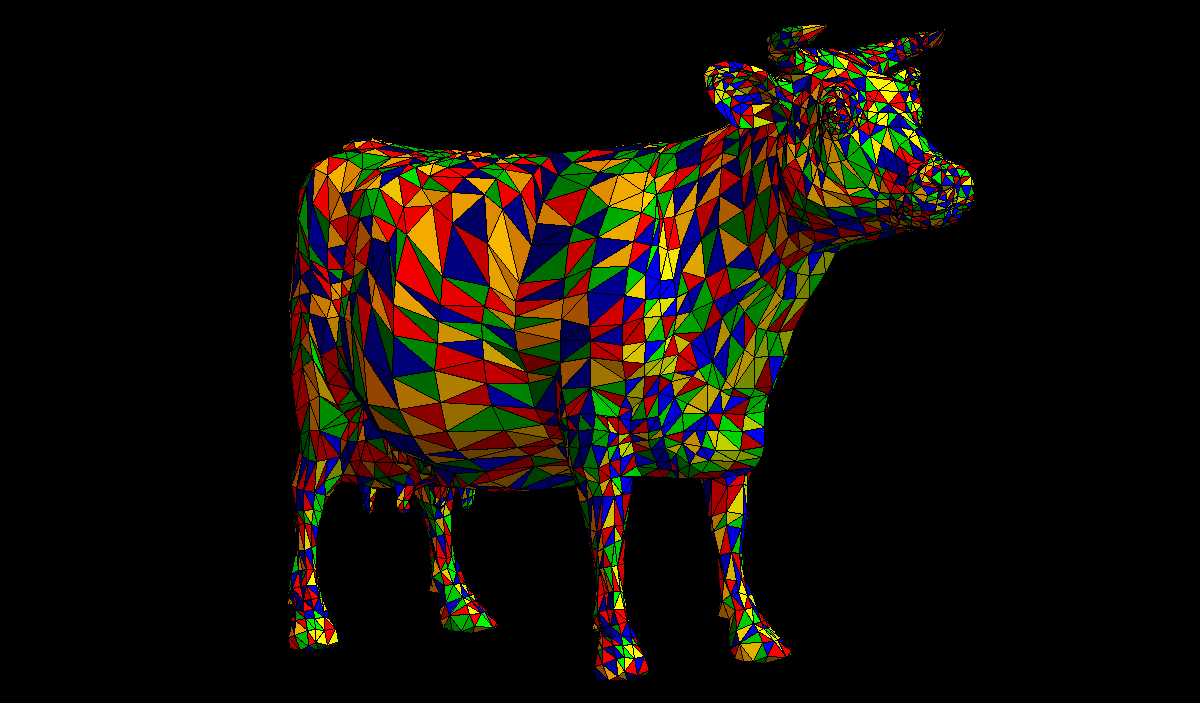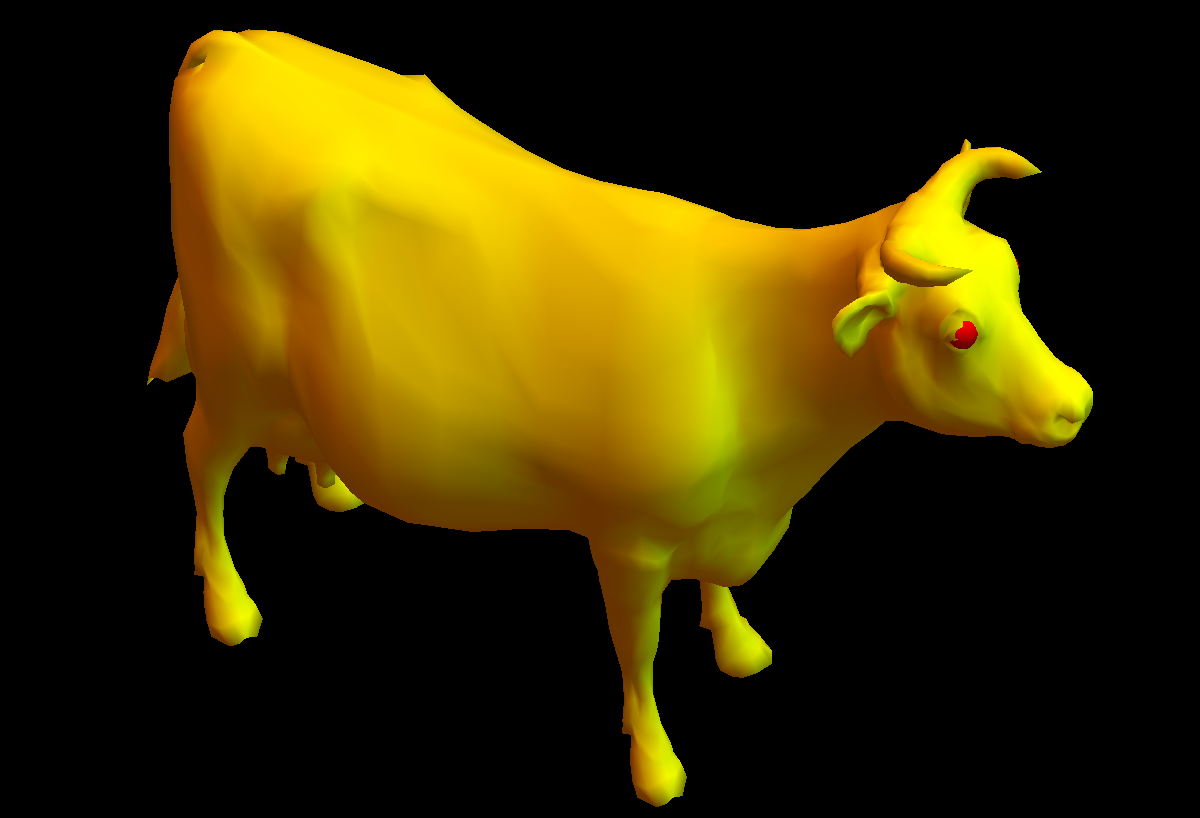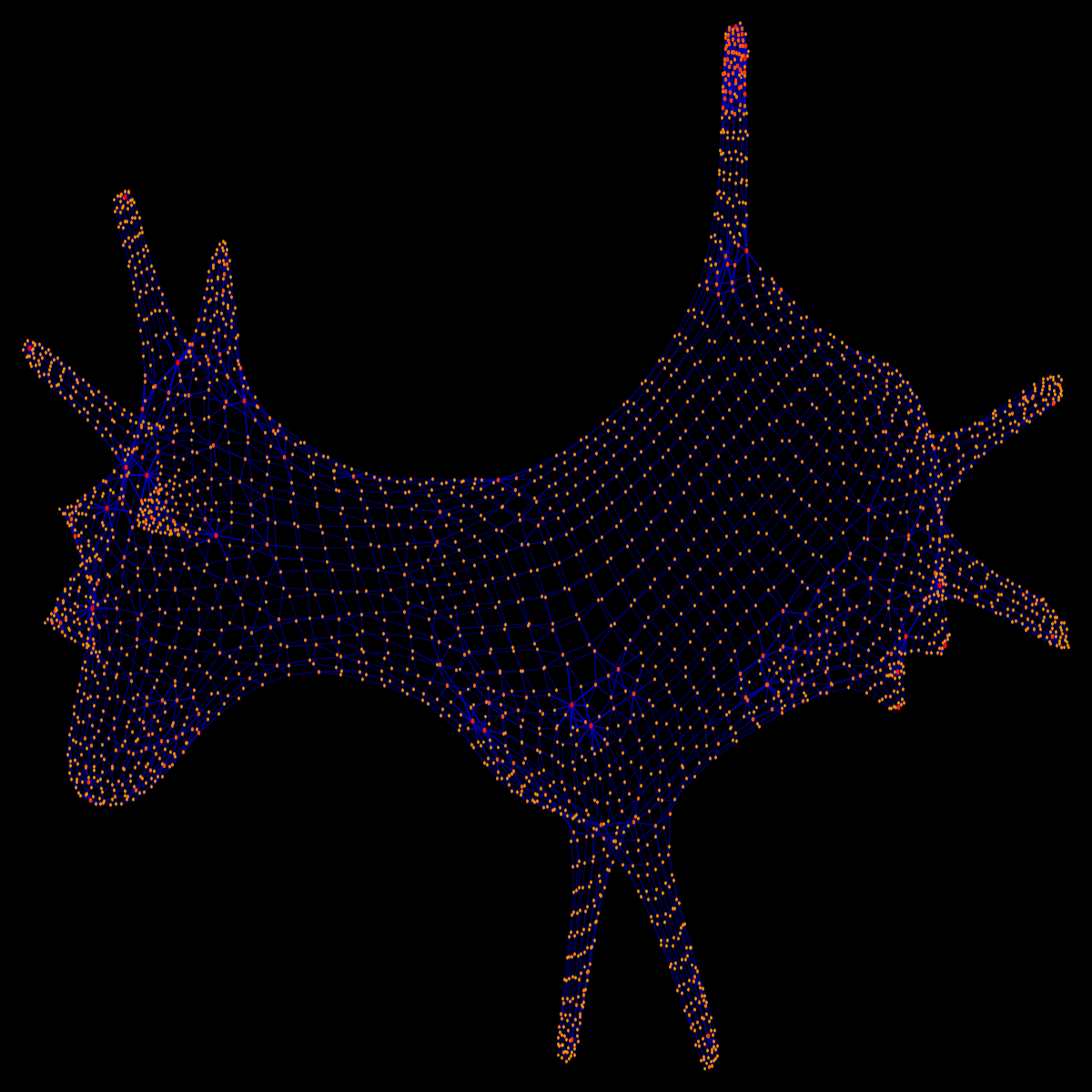# The cubistic cow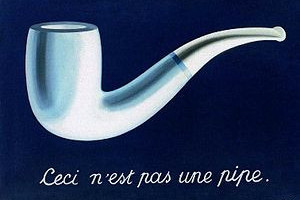As some of you knew (and acknowledged in the exam), the last problem in the final exam referenced to the the treachery of images. Below are more pictures of the cow. The third one is the graph of the mesh alone.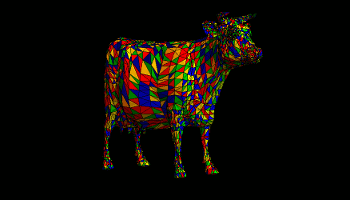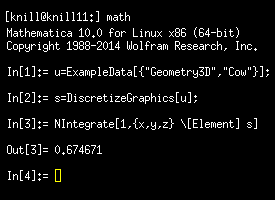By the way, the story of the cow was motivated by a new feature of Mathematica 10, which now allows to integrate over objects like cows. Here is an example (which unlike in the Mathematica documentation does not require you to open a Mathematica notebook but can be done direct computation with the kernel. You have typed this before the GUI has only started up. But graphical user interfaces are for pussies anyway. ```u=ExampleData[{"Geometry3D","Cow"}]; s=DiscretizeGraphics[u]; NIntegrate[1,{x,y,z} [Element] s] ```